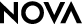Three Men and a Balloon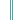Student Handout

At Arm's Length

While they are in the air, balloonists often need to determine how far they are from a specific location or object in the distance. To do this, they can rely on high-tech equipment for exact measurements, like the balloonists in the program, or they can estimate distances using their arm lengths, as some amateur balloonists do. Try it and see.

Part I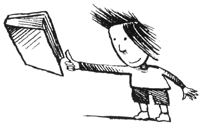1. Choose a target that is about four to five inches wide. At arm's length, hold your thumb up next to the target. What do you notice about the width of your thumb compared to the width of the target?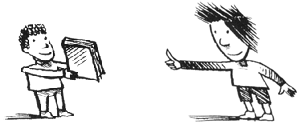2. Now, have your partner hold the target up while you move away from it. Hold your thumb up at arm's length, close one eye, and align your thumb with the target. Move closer or farther until the width of your thumb appears to be the same size as the width of the target. Notice how far away you are from the target, and mark your position. Measure the distance you are from the target and record that number on the chart.

3. Next, change positions until it takes two thumbs to equal the width of the target. Again, notice the distance you are from the target, and mark your position. Measure and record the distance.

4. Finally, move until one-half thumb equals the target. Mark your position. Measure and record the distance.

5. Continue this process until you have filled in the chart.

6. Now have your partner do steps 1-5. Then answer these questions together before going on to Part II:

How does the relationship between the width of your thumb and the width of the target change as you get closer to the target? How about as you get farther away?

 Number ofthumbs widthsacross target 3 2 1 1/2 1/3 Distancefrom targetPart II

A simple formula can be used to estimate your distance from a target when your thumb appears to be the same width:

d (estimated distance) = N (number of thumbs per target) x A (arm length)

To use this formula, you and your partner will need to make the following measurements and calculations. Take turns doing each measurement.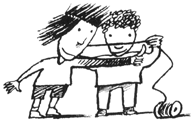1. Measurement A:

Hold your arm out as shown, and have your partner use a length of string to measure the distance from your eye to the top of your thumb. Measure the string and record the result.

A = ______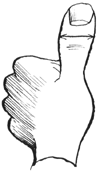2. Measurement B:

B =______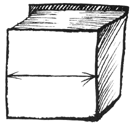3. Measurement C:

Use string to measure the widest part of your original target. Measure the string and record the result.

C = ______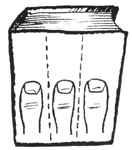4. Calculate N:
Relationship Between Your Thumb and the Target

Calculate how many times your thumb fits into the target if you are standing arm's length from it (N = C / B). Record the result.

N = ______

5. When all of the measurements have been taken, use the formula to estimate the distance you would be from the target if your thumb and the target appear to be the same width. Compare this estimate to the distance you recorded in the chart.

Estimated distance: ______

Actual distance: ______

6. This formula is useful when you are at a distance where your thumb appears to be the same width as the target. How can you estimate distances when more than one thumb or less than one thumb appears to fit into the target? (Think about how the distance to the target changed when your thumb appeared to be twice as wide or half as wide as the target.) Use the data on your chart to help you change the formula so that it can be used to estimate any distance.

-->